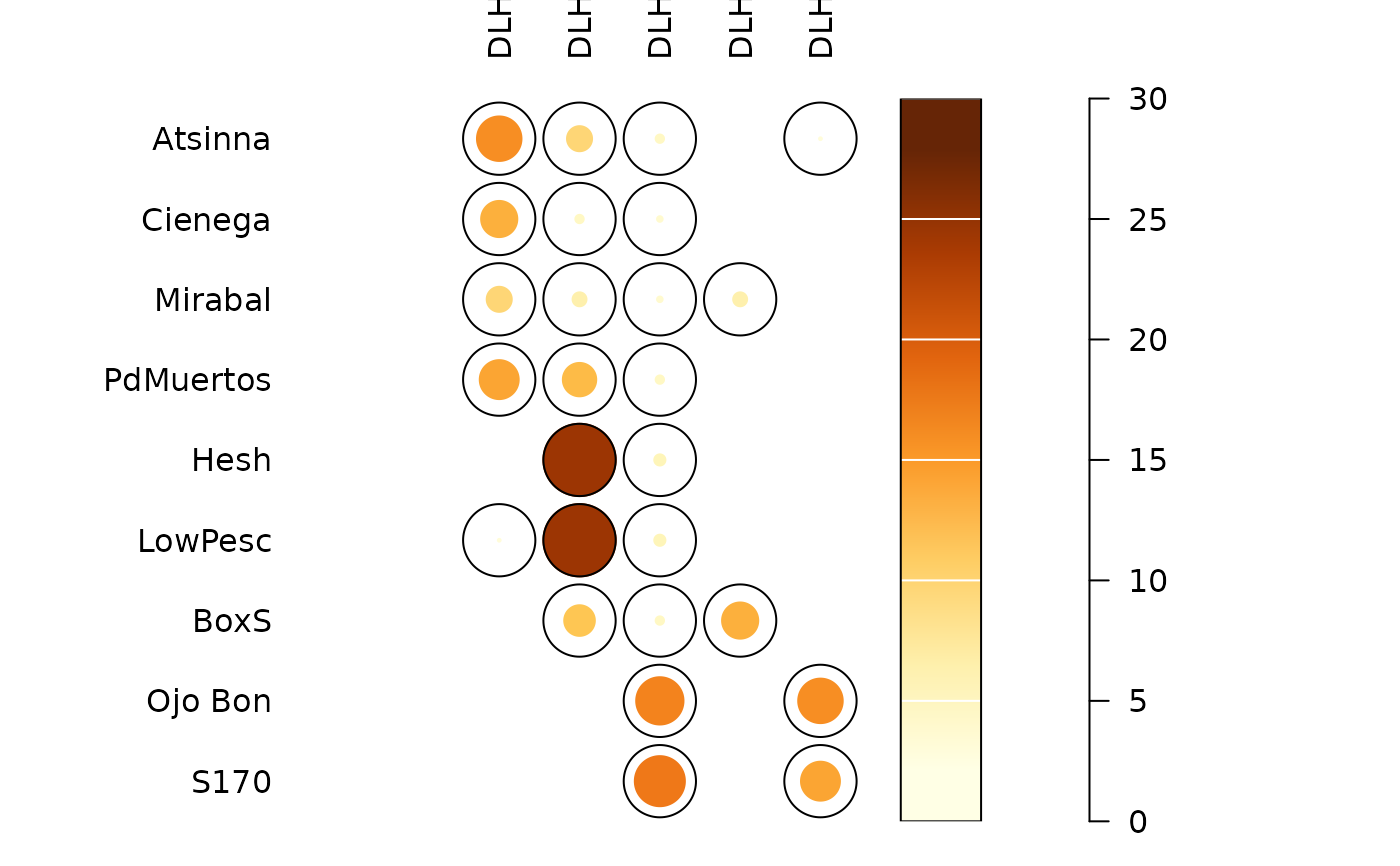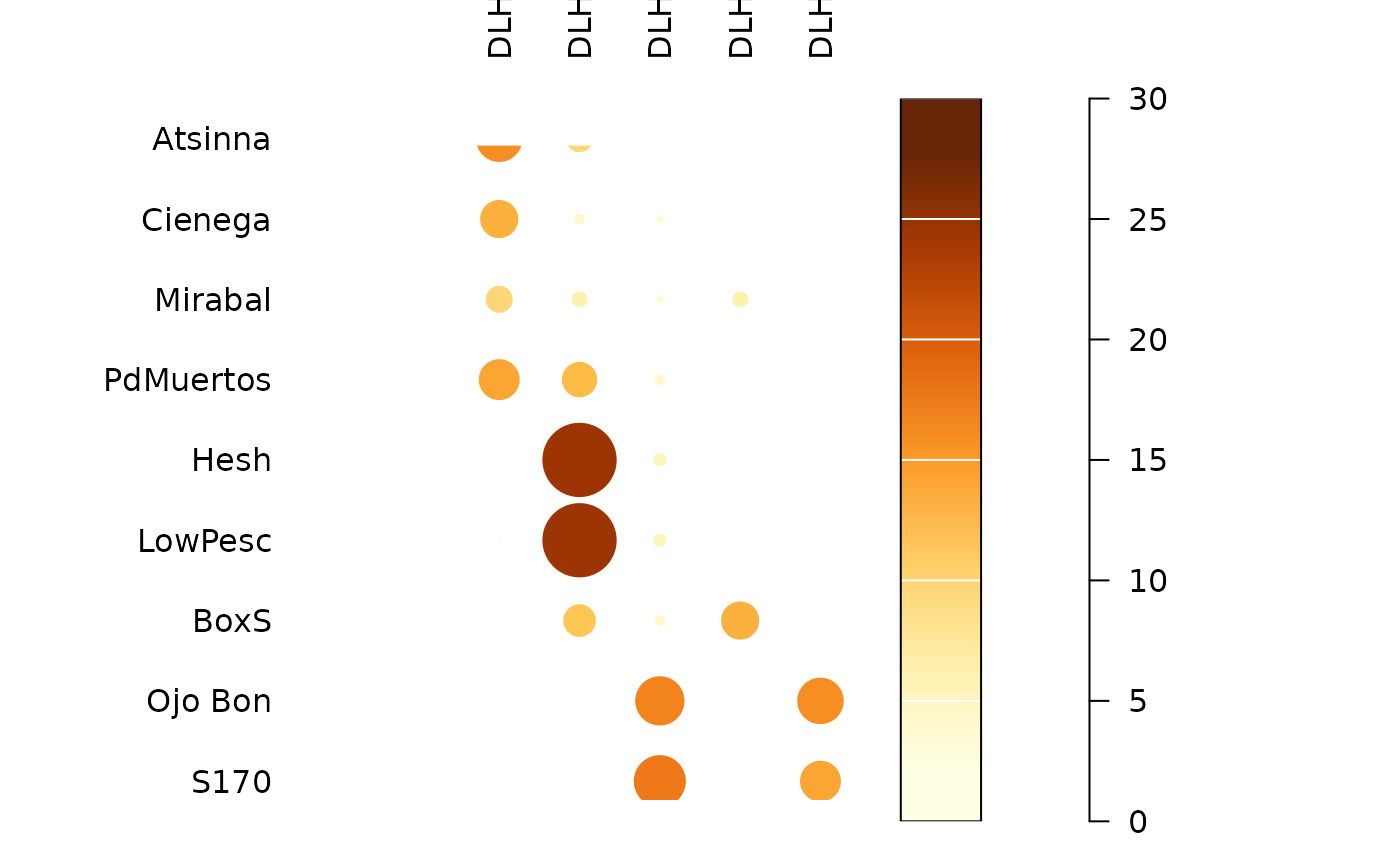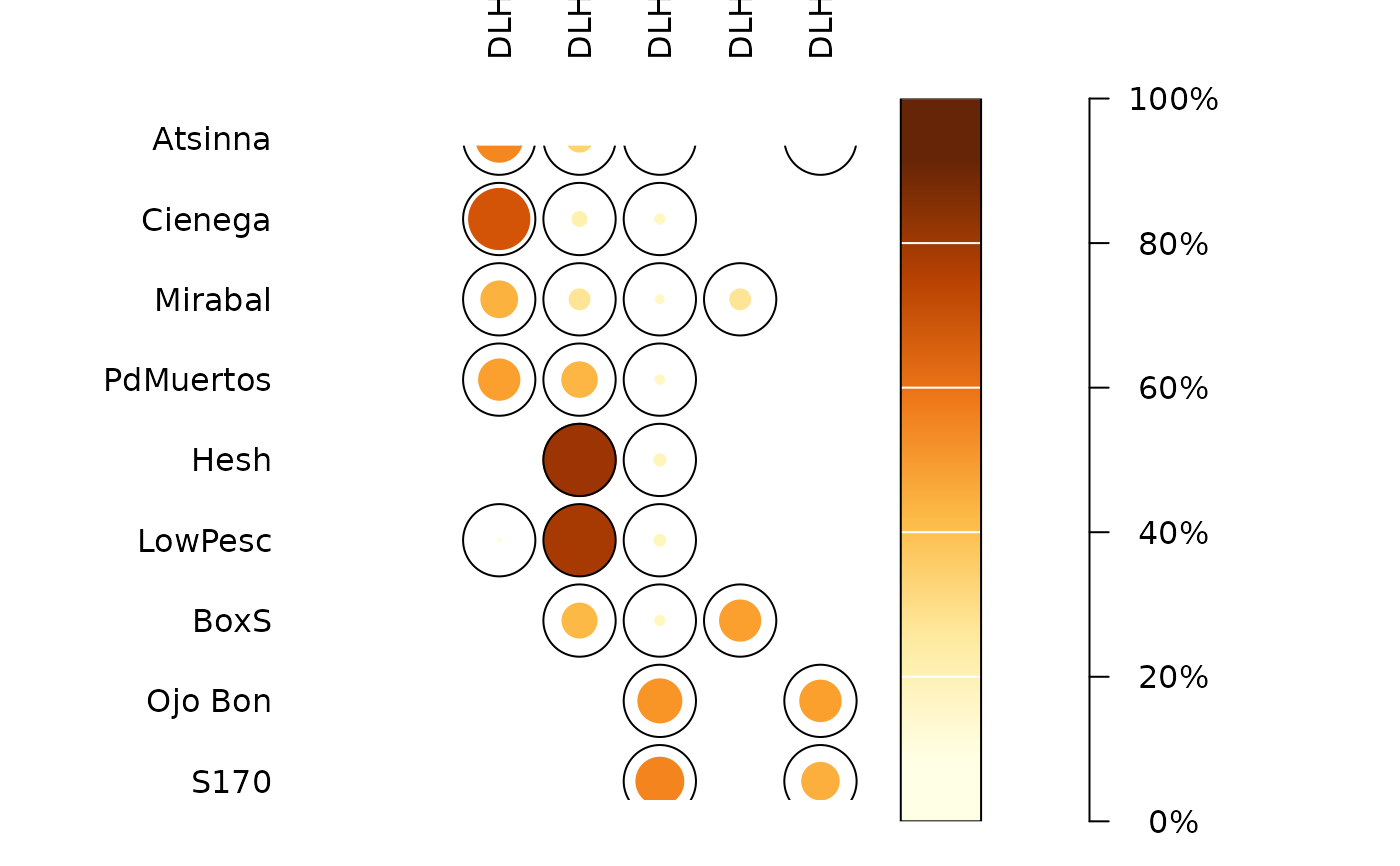Plots a spot matrix.

plot_spot(object, ...)

# S4 method for matrix
plot_spot(object, threshold = NULL, diag = TRUE, upper = TRUE, ...)

# S4 method for dist
plot_spot(object, diag = FALSE, upper = FALSE, ...)

# S4 method for OccurrenceMatrix
plot_spot(object, diag = FALSE, upper = FALSE, ...)

## Arguments

object An abundance matrix to be plotted. Extra parameters to be passed to threshold. A function that takes a numeric vector as argument and returns a numeric threshold value. If NULL (the default), no threshold is computed. A logical scalar indicating whether the diagonal of the matrix should be plotted. A logical scalar indicating whether the upper triangle of the matrix should be plotted.

## Value

A ggplot2::ggplot object.

## Details

The spot matrix can be considered as a variant of the Bertin diagram where the data are first transformed to relative frequencies.

## Note

Adapted from Dan Gopstein's original idea.

Other plot: plot_bar, plot_diversity, plot_line, plot_matrix

N. Frerebeau

## Examples

## Plot spot diagram of count data...
data("mississippi", package = "folio")
counts <- as_count(mississippi)

### ...without threshod
plot_spot(counts)### ...with the  column means as threshold
plot_spot(counts, threshold = mean)### ...with the column medians as threshold
plot_spot(counts, threshold = median)